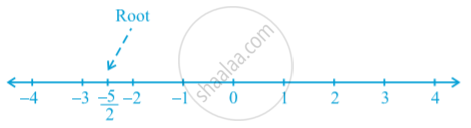# Linear Equation in Two Variables

## Notes

Consider the following equation: 2x + 5 = 0
the root of the equation is  -5/2. This can be represented on the number line as shown below:While solving an equation, you must always keep the following points in mind.
The solution of a linear equation is not affected when:

1. The same number is added to (or subtracted from) both sides of the equation.
2. You multiply or divide both sides of the equation by the same non-zero number.
So, any equation that can be put in the form ax + by + c = 0, where a, b and c are real numbers, and a and b are not both zero, is called a linear equation in two variables.

## Notes

Linear equation is an algebraic equation having a degree of 1. We can say 2x+5=0 is a linear equation, as the degree of the variable x is 1. But in a linear equation in two variables have two variables. E.g. 2x+5y-1=0 is in the form of a linear equation with two variables, x and y. ax+by+c=0 is a standard form of linear equation in two variables, where a is the coefficient of x, b is the coefficient of y, and c is a constant term, a, b and c are real numbers, also a and b are not equal to zero.

In this chapter, we have to learn about the Pair of Linear Equations in Two Variables. Generally, A Pair of Linear Equations in Two Variables are written as a_1x+b_1y+c_1=0 and a_2x+b_2y+c_2=0. Example 2x+9y+12=0 and 6x+1y+8=0
To learn how to solve A Pair of Linear Equations in Two Variables, there are two methods: Graphical and Algebraic. We will study this in the following concepts.

If you would like to contribute notes or other learning material, please submit them using the button below.

### Shaalaa.com

Pair of Linear Equation in two variable part 1 (Introduction) [00:12:13]
S
0%